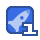# 同等学力_在职研究生考研_离散数学&组合数学2007-201805真题及答案.pdf52收藏

2005年数学 第一部分数学基础课程 (共40分) 、形式化下列语句(共3分) 1.(1分)有且有一个太阳 s答:全域:全部天体P(×)表小x为太阳;Qxy)表示x与y相等,则:彐vyP(x)∧(P(y)→Qxy 原答:P∧q,其中,p:有太阳,q:有一个太阳 2.(2分)任意两个相异实数xy之间必可找到另一个实数z. S答:全域:全体实数R(x):x为实数则原句可形式化为 vxy(R(x)AR(y)∧x≠y→2(R()A(x<zy∨y<x) 或设R(x:x为实数Nxy):x≠yG(x2,y):x<z<y则原句可形式化为: vxvy(R(x)AR(y)∧N(xy)→彐z(R(z)∧(G(x,z,y)VGy<zx) 原答:R(x):x是实数E(zy)X=yL(xy:x>y ↓xy(R(x)∧R(y)∧-Eⅸxy)彐z(R()∧L(x,2)∧L(z,y))∨(R(z)∧L(yz)∧L(z,x) 二、填空题(共9分 1、(2分)设A-[1,2,3,4],则在A上的二元关系共有216个;其中有15个是等价关系。 答:2 16 1)集合A元素个数|A|=4,所以A上元关系的个数为24×4=216=65536个。 2)因为等价关系与集合划分一一对应,所以找出集合A的所有划分,每一个划分对应一个等价关系,共15个 划分的子集对应于等价关系的等价集 划分成一个等价集(1个等价关系{1,2,34} 划分成两个等价集(7个等价关系:{1,23}{4}{12,4}3}/1,34}{2}2,34{1}/{1,233,4}13}{24}/1,4}{2,3} 划分成三个等价集(6个等价关系):{1,23},4/13}2{4/(14,23/12,34/(1}{24}3}/(1234 划分成四个等价集(1个等价关系:{1{2{3}4} (注:等价关系:设R是非空集合A上的二元关系,若R是自反的、对称的、传递的,则称R是A上的等价关系。) n(n+1) 2、(1分)设|A|=n(即集合A的基数为n),则在A上有2-2个不同的对称关系。 答:设集合A的基数A为n,在A上有2n2-个不同的自反关系,有22个不同的对称关系。 解析:A上的关系可以用一个nxn的关系矩阵表示,自反关系只要求对角线上的元素为1,其他位置上的元素任意, 可以0或1,因此A上的自反关系个数为2-n种。A上的对称关系个数为2_2种。 4 3、(2分)(a+b+c)的展开式经过合并同类项后有C(64)=15项。 原答:共C=15项 (x1+x2+x3-4的非负整数解) s答:三项式可以将其中两项合成整体,用二项式定理来处理:(a+b)=C0an+Cnan-1b1+…+chan-kbk+…+Cmbn (a+b)+c)4=C9(a+b)4+C(a+b)3c1+C(a+b)2c2Cac3+Ctc4 a+b)4共5项 (a+b)3=Cga3+C3a2b1+C3a1b2+C3b3共4项 (a+b)2共3项 (a+b)共2项 共5+4+3+2+1=15项 4、(2分)标有1、2、3、4的四张数字卡片,要求数1不排在千位上,数2不排在百位上,数3不排在十位上, 数4不排在个位上,那么用这四张卡片组成的满足要求的四位数共D4=9个。 答:考完全错排D4=4(1 1!2!3 624 树形图——枚举法 第4题的树形图 解:2143,2341,2413,3142,3412, 3421,4123,4312,4321 5、(2分)以三种不同的颜色来给某房间的四个墙壁着色,房间的地面为长方形(如下图所示),每个墙壁只着 一种颜色,任何相邻的两个墙壁的颜色都不同,共有18种着色方案。 e 答:利用容斥原理 A:表e1和e2同色,B:表e2和e3同色,C:表e3和e4同色,D:表e4和e2同色 A∩B∩C∩D|=|S|-|A∪BUc∪D|=|S|-|A|-|B|-C|-|D|+|A∩B|+|A∩c|+|A∩D|+|B∩c|+|B∩D|+|C∩D|-|A∩Bnc| A∩B∩D|-|AnC∩D|-| BnCnD|+|A∩BnC∩D 34-4×33+6×32-4×3+3=81-108+54-12+3=18 若不允许用2种颜色,要减去6种方案。 第5题的树形图,18个解 如果不允许使用2种颜色,还需要减去以下6种 三、问答题(6分) 有r个正方形排成一行,今用红、黄、白、蓝四种颜色给这个r个正方形染色,每个正方形只能染一种颜色, 如果要求染红、黄、白色的正方形分别至少出现一个,问有多少种不同的染法? 答:考指数型母函数 Ge(x)=(1 +- 3! e(e-1 3e3x+3e 3∑ k! +322=012kxk-∑ k kl 3k+1+3:2k-1) 方案数ar=47-37+1+3·27-1 四、证明(共22分) 1、(3分)下列等值式是否正确,如正确请证明,如错误请举出反例。 (vx)(yP(x)∧Q(y)→S(x,y)=-(×)y(P(x)∧Q(y)∧aS(x,y) 解:正确,推理如卜 (v×)(vy)(P(x)∧P(y)→Q(xy) =((x)(Ey)(P(x)APly))-Q(x,y)) 量词辖域收缩等值式 ((3x)(3y)(P(x)AP(y))vQ(xy)蕴含等值式 (x y)(P(x)A P() Q(x,y)) (x)(3y)(P(x)AP(y)∧-Q(xy)德摩根等值式 (注:同2011年四-1) 2、(3分)设f:RXR→R,f(xy)=X+y; g:R×R→R,g(xXy)=Xy 证明:(1)f是满射的,但不是单射的 (2)g是满射的,但不是单射的 证1 (1)对于任意的b∈R,存在<0b>∈RR,满足f0.b)=0+b=b因此f是满射的。但由于f(0,1)=f(1,0)=1,因此f不是单射的 (2)对于任意的b∈R,存在<1b>∈RXR,满足g(1b)=1×b=b因此g是满射的。但由于g(,1)=g(1,0)=0,囚此f不是单射的 证2:(1)因为对于任意B∈R,皆存在<xy>∈R×R,使得fx,y>=x+γ=B成立,根据概念,f是满射的。 但从x+y=B中,并不能确定唯一对应的<xy>,故f不是单射的。 (2)因为对于任意B∈R,皆存仁<xy>∈RR,使得fxy>xxy=B成立,根据概念,f是满射的。 但从ⅹ×y=B中,并个能确定唯一对应的<xy>,故f不是单射的。 3、(4分)设G是一个有n个结点m条边的连通简单平面图,若n≥3,则m≤3n-6. 证明:设G有k(k≥1个连通分支,若G为树或森林则m=nk≤3n6(n≥3),若G不是树也不是森林,则G中必含圈 又因为G是简单图所以各圈的长度均大于或等于3,因各面次数至少为1(≥3)又2=1+乙2在13时达到最大值 4、(4分)证明:任何连通简单平面图至少有一个结点的度数不超过5 证1:假设每个结点度数都大于6,则∑d(v)>=6n,由握手定理可知:2m=d(v;)>=6n 因而m≥3n,G是简单平面图m≤3n-6即m+6≤3n,与上题结论矛盾,问题得证。 证2:若G的阶数n≤6,结论显然成立,n≥7,若最小度8≥6,由握手定理可知:2m=∑rd(v)>=6n。 因而m≥3n,与上题结论矛盾,问题得证。 5、(8分)给定群<G,o>,且a∈G定义映射f(x= aoxoa-1,x∈G.证明:f是<G,o>到其自身的同构映射。 证1:显然有f:G→G,下面证明f的满射性与单射性。 对任意y∈G,存在a-1oyoa属于G,使得f(a-1oyoa)=a(a1oyoa)oa1=y,因此f是满射的。 假设f(x)=fy),那么有a-1oxa=a-1oyoa,根据群的消去律,必有ⅹ=y。因此f是单射的 最后验证f为同态映射。对于任意x,y属于G f(xoy =a o(xoy)o a -1 =aoXoyo a-1 =(aoxo a-lmaoyo a 1)=f(x) of(y) 证2:对于x,y∈G有f(xoy)=a(xya-1=(aox0a-1) o( oa-1)=f(x)of(y)所以f是G的自同态。 任取y∈G,则a-1oyoa∈G,且满足f(a-1oyoa)=ao{a-1oyoa)ca-1=y所以f是淸射的。 假若fx)=f(y),即a-oxoa=a-oyoa,由G中的消去律必有x=y,从而f是单射的。 综上所述,f是<G∞>到其自身同构映射 2006年数学 第一部分数学基础课程 共40分) 、形式化下列语句(共4分) (1分没有不犯错误的人。 答:设M(x):ⅹ是人。Q(x):x犯错误。形式化为:(x)(M(x)→Q(x) 2.(2分)虚数既不是有理数也不是无理数。 答:设W(x):ⅹ是虚数。P(X):ⅹ是有理数。Q(x):x是无理数。形式化为:(v×)W(x)入_P(x)∧_Q(x) 、填空题(共9分) 1、(3分)设集合A={ab,c,A为A上的恒等关系,EA为A上全域关系 1)设写出EA-IA Isa, b>, <a, c>,<b,a>sb, c><C, a>,<c, b> (2)A上含有序对个数为4或5的二元关系数目最多,这样的二元关系数目为_C(94)=C(95)=126 t4*T:(1) EA<a, a>, <a, b>, a, c>, cb, a>, <b, b>, <b, c>, <c, a>, <c, b>, <c, c>), IA=Sa, a>, <b, b>, <c, c>l (2)因为在A上的任何二元关系都是A×A的子集,而AXA共有9个元素, 共可组成C9+C+…+C-=29个了集,C=C最大,因此含有序对个数为4或5的二元关系数目最多为C4=126。 2、(2分)把6个人分成两组,每组至少2人,共有C(62)+C(63)/2=25种不同的分组方式。 解析:有两种分组方案,①一组2人,一组4人,②两组都是3人,故共有C2+C3/2=25种不同的分组方式 4 3、(2分)(x+2y+3z+4w)展开式经过合并同类项之后,xy硎w的系数为4*2*3*4=576 解析:由多项式定理 (+x2+…+x)=∑( x, nix, n2 12…n2 10001000 0101/50101/5 ,其中 001010010 nn2…n2 H1h2!n,! 00000000 721+12+…+nt=1。故x2(2y)2(3z)14W1的系数为4!=24。XzW的系数为24*24=576 4、(2分)从1到30的正整数中任意选取三个不同的数,使得它们的和能被3整除,则共有3c103)+C(10,1)3=1360 种不同的选取方法。 解析:1,2…,30这30个数可以分成三组:被3整除者为一组,除以3余1者为一组,除以3余2者为一组,显然 每组有10个整数。如果三个整数都选自同一组,其和必能被3整除,如果每组选取一个整数,其和也必能被3整除。 因此选取方法总数为:C(10,3)+C(10,3)+C(10,3)+103=1360 三、解答题(共11分) 1、(3分)设P↑Q=—(P∧Q),试将-P,P∧Q,P∨Q仅用联结词↑表示出来。 解析:P↑Q表示P和Q进行先与后非的操作。 TP=P↑P p∧Q=(P↑Q)↑(P↑Q) p∨Q=1P↑1Q=(P↑P)↑(Q↑Q) 2、(8分)在信道上传输仅由上而下个字母ab,cd,e组成并且长度为n的词,规定连续出现两个a的词不允许传输, 用hn)表示这个信道上允许传输的长为n的词的个数 (1)求h(1)h(2); (2)建立关于h(n]的递归关系; 3)求h(n)的通项公式。 原解:h(n)表示信道上允许传输的长为n的词的个数则h(1)=5,h(2)=5*5-1=24 令n>=2如果单词第一个字母是b或cd,e,则余下长度n1的位置上仍然是在相同限制条件下对ab,c,d,e这5类字 母的排列,排列方法为hn-1)如果单词第一个字母是a那么第二个字母就是b或cde 如果第二个字母是b,则该单词可以有h(n2)种方法构成,如果第二个字母是c或d,e,同样该单词也可以有h(n2)种 方法构成。于是有h(n)=4h(n1)+4h(n-2)n>=2该递推关系的特征方程x24×4=0的根为2+2√2和2-22,即 h(n)=c1(2+2√2)+c2(2-2√2)利用h(1)=5,h(2)=5*5-1=24可解得 h(n) 4+3√2 (2+2√2) 3√2 8 (2-2√2) 类似的题:在信道上传输仅用3个字母a,b,c组成并且长度为n的词.规定连续出现两个a的词不能传输.试确定 这个信道允许传输的词的个数 T解:令hn)为允许传输长度为n的词总数,n=1,2,…,直接计算知,h(1)=3,h(2)=8 设n≥3,第一个字母是b或c的词数均为h(n1);第一个字母是a的词,第二个字母必须是b或c,这种词的数目为2h(n2) 故有以下递归关系:h(n)≥=2h(n-1)+2h(n-2,n=3,4..且h(1)=3,h(2}=8 该递归关系的特征方程为x2-2x-2=0其特征根为q1=1+√3,q2=1-√3 故一般解为:hn)=c1(1+√3+c2(1-√3)n,n=3,4 由边界条件h(1)=3,h(2)=8解方程组 c1(1+√3)+c2(1-√3) 得 2+√3 lc;(1+√3)2+c2(1-√32=8 hn)23(1+√3)+-23(1-y 四、证明题(共17分) 1、(3分)设A={<a,b>|a,b为任意正整数},A上的二元关系,R={<<a,b>,<cd|ad=be证明R是A上的等价关系。 d>∈dd-bcsa/b-c!d 任取<b>属于A,a,b是正整数,那么有 ab=ab→xa,b>,<a,b>∈R,R是自反的。 任取<ab>,<c,d属于A,有 <<n,b>,<,d>>∈R=uhb=chcl=h→<db,anb>>∈R R是对称的。 任取<b>,<;d,/属于A,有 <b>,<c,db>∈RA<<C,d>,<f>∈R→uhb= cld acid=ef W/b=e-<<,b>,</>∈R,R是传递的, 让2:①任给<Xy>∈A有xy=yx→<xy,<xy>>∈R,即R是自反的。 ②)仟给<xy>,<,q∈A若<<xy>,<p,a>∈R则xg=yp,可得py=qX→<p,q>,<xy>>∈R因此R是对称的。 ③任给y>,≮q>,<m,n>∈A若<xy>,<pq>>∈R且<p,q>,<mn>∈R 则Ⅺ=yp,pn=qmPM2+3(11)=3→xn=m→<xy>,<m,n>∈R此R是传递的 因为R是自反的,对称的,传递,因此R是A上的等价关系。 2、(4分)设G是一个简单图,G的每个顶点的度数至少是3。证明图G中一定存在长度为偶数的图。 解析:对简单图G的结点个数n进行数学归纳证明:当n=4时,G为完全图,结论显然成立所得的圈的长度为4. 设当n=k时结论成立,长度为偶数的圈为C。则当n=k+1时,长度为偶数的圈C也在结点数为k+1的图中,因此结论成立 3、(4分)设G是一个简单连通图,G是G的子图,而且|G|<|vG川。证明G中必然存在这样一条边巳 e的一个端点属于G,另一个端点不属于G'。 解析:用反让法,由于|VG|<|VG川,不妨设点集Ⅴ(S)=V(G)√(G'),假设G中不存在一个端点属丁G, 另一个端点不属于G'的边e,那么图G可分成两个互补连通的子图S和G,这与G是简单连通图相矛盾。因此假设 不成立,G中必然存在这样一条边e,e的一个端点属于G,另一个端点不属于G。 4、(6分)设H,K是有限群G的两个子群,e是G的单位元,这两个子群的阶分别为H|=m,|K|=n 如果m与n互素,那么H∩K={e} 解析:因为HK是有限群G的了群,那么必有G的单位元e∈H且e∈K,即e∈H∩K。不妨设存在与e相异的 元素a也属于H∩K,令|H∩K弌t>=2,由于H∩K是H和K的子群,根据拉格郎日定理tm且tn,这与条件m与n 互素相矛盾。故元素a不存在。|H∩K|=1,即H∩K=e}(参考《离散数学》教材,第十章24题) 2007年数学 第一部分数学基础课程 (共40分) 、用逻辑符号表达下列语句(每小题2分,共4分) 分别用两种量词形式写出:在北京居住的人未必都是北京人 S答:全域:所有人Px表小x居住在北京:Q(x)表小x为北京人,则: 全称量词表示为:x(P(x)→Q(x)存在量词表示为:(P(x)→-Q(×) 2.不存在比一切实数都大的实数。 T答:全域:所有数R(x表ⅹ是实数M(xy)表xy (1)任何一个实数,都存在另一个实数比它大:YxyR(x)∧R(y→M(xy) (2)不存在比一切实数大的实数:=(xvyR(x)∧Ry)∧M(yx) 、填空题(共10分) 1.(4分,每空1分)设A={a,b,c},B={1,2} B4中可以定义(8)个函数,其中有(6)个满射函数。 AB中可以定义(9)个函数,其中有(3)个不是单射函数。 2.(2分,每空1分)一个有v个顶点E条边和f个面的平面图的邻接矩阵是(n)行(n)列的矩阵。 3.(2分)由2个x,1个y,2个z共5个元素组成的不同排列的总数是(5/2!2130)。 4.(2分)能除尽600的正整数有(4*2*3=24)个。 解析:600=23×3×52=2Ⅺ×3x2×5230≤x1≤30≤X1≤10≤X1≤2则4*2*3=24 三、计算题(共14分) 1.(4分)计算(P→Q)4(P→_Q)的主析取范式,并分别给出该式为真和为假时,P、Q的赋值。 2(5分)求由八个相异元素a1,a2,a3,a4,a5,a6,a7,aB作成的全排列中只有四个元素不在原排列位置上的排列数, (这里a1,a2,a3,a4,as,a6,a7,ag是指原排列)。 8x7×6×5 111 x4x1- +]=630 I!2!3!4! 3.(5分)n个完全一样的球放到8个不同的盒子里(n≥8),不允许有空盒,问共有多少种不同的组合方案? (用母函数的方法)。 答 G(x)=(x+x2+x3+)= n+8-1 1-x)=x 开=0 n+8-1-8 8 n-8 四、证明题(共12分) 1.(4分)设R是集合A上的二元关系,试证明:如果R是自反的,并且是传递的,则合成关系R?R满足RR=R 证任取<,b>, <n,b>∈ReR→c∈A(4,c、∈RAC,b∈R) <a,b>∈R(R的传递性) 于是 ROCk 反之,任取<b>, ,b>∈R→<,o>∈RAn,b∈R(由十R自反) →<b>∈R。R 于是RRR 综上所述,命题得证。 2.(8分)设G是有n3个顶点的简单连通图,且G的最小度8≥,试证:G是汉密尔顿( Hamilton)图。 8/ 2008年数学 第一部分数学基础课程 共40分) 用逻辑符号形式化下列语句(本大题共2小题,每小题2分,共4分) 每个人的指纹都不相同。 答:设M(x):x是人;N(x,y):x≠y,即x与y是不同的人;E(x,y):x与y的指纹相同。 则原句可形式化为以下两种形式之 ·①VxyM(x)∧M(y)∧N(x,y)→E(x,y) ②2(x)(M(x)→(vy)(M(y)∧Y(x,y)→-E(x,y)) 说明:如仅缺少N(x,y)的内容,则只给1分。 2.自然数不是奇数就是偶数,且奇数不能被2整除。 答:设P(x):x是自然数,Q(x):ⅹ是奇数,R(x):ⅹ是偶数,D(x):ⅹ能被2整除。 原句可形式化为 yx((P(x)→(Q(x)∨R(x)∧(Q(x)→_D(x)) 说明:(1)如仅答对部分内容最多给1分。 (2)全句必须写成一个式子,且中间用联结词∧联结,否则扣0.5分。 二、填空题(本大题共4小题,第1小题每空1分,第2、3、4小题每空2分,共10分) 1.设A、B均为有穷集合,A和B的基数分别是m和n(m>0,n>0)。 (1)当m和n满足m=n时,存在从A到B的双射函数。此时共可生成n!个不同的双射函数。 (2)当m和n满足皿≤n时,存在从A到B的单射函数。此时共可生成Cmml!=Am个不同的单射函数。 2.已知5位老师和3位学生围圆桌就座,如果要求学生两两不相邻,则有4!·P(5,3)=4!·5·4·3或140种就座方案。 3.整除2310的正奇数有24=16个 提示:2310=2×3×5×7×11,2310的奇因子是357114 的形式,其中a,b,C,d=0,1 4.设图G的顶点集合为v(G)={v1,v2,w3,V小,边集合为E(G)={vv2,v2v3,v3v;V1,v1v}则G的生成树有8棵。 三、解答题(本大题共3小题,第1、2小题每题4分,第3小题8分,共16分) 1.设P↓Q=-(PVQ),仅用联结词↓分别表示出_P,P∧Q,PVQ。 解:(1)-P台-(PVP)台P↓P 分 (2)P∧Q 台-(-P∨-Q 台(-P)4(-Q 台(P↓P)↓(Q↓Q) (见下面说明) (3)P∨Q 台((P∨Q) 台-(P+Q) 台(P↓Q)↓(P↓Q 如(2)、(3)小题中仅做对一题则给2分,(2)和(3)小题都做对则给3分 2.设T是一棵有13个顶点的树,称树中度为1的顶点为叶子。如果T的顶点的度只可能是1,2,5且T恰好有3个 度为2的顶点,那么,T中有多少个叶子? 解:设T中有x个叶子,则T中有13-3—x=10—X个度为5的顶点 由于树中的边数等于顶点个数减去1,即边数为12 分 由顶点度数之和等」边数的两倍得 1*x+2*3+5*(10-x)=2*12 解得x=8,故T中有8个叶子 2分 /28 3.求1,4,5,8,9这五个数字组成的位数的个数,要求4,8出现的次数均为偶数,而1,5,9出现的次数不加限制。 3.解设满足条件的i位数的个数为a,则序列a1,a2a3L对应的指数型母函数G(x)为 G(x)=(1+++L)2(+x++,+L) 2分 2 由于e2=1+x+ L故e-x=1 L 2!3! 于是1+ x+x+1 -3分 2!4! 2 故Gx)=( De=-(e+2e+e) (5+2.3+1) 2分 故 (5+2.3+1) 1分 四、证明题(本大题共2小题,第1小题4分,第2小题6分,共10分) 1.设R是非空集合A上的二元关系,R满足条件 (1)R是自反的 (2)若<a,b>∈R∧<a,c>∈R,则<b,c>∈R 试证明R是A上的等价关系。 证明参考《离散数学》第七章,39题 证明:由条件(1),R已满足自反性。需证明R满足对称性和传递性。 1)对于任意的<a,b>, <a,b>∈R且由条件(1) =><a,b>∈R∧<a,a>∈R 分 由条件(2)=><b,a>∈R -1 分 所以,R满足对称性。 2)对于任意的<a,b>,<b,c ,b>∈R∧<b,c>∈R 由对称性<b,a>∈R∧<b,c>∈R 1分 由条件(2)<a,c>∈R -1分 所以,R满足传递性。综合1),2)可得,R是A上的等价关系。 2.随意地把一个9×3棋盘的每个方格涂成红色或蓝色,求证:必有两行方格的涂色是一样的。 证明:用红、蓝两色去涂1×3棋盘,共有23=8种涂色方法。 分 设a1(i=1,2,L,8)表示第i种涂色方法.设J是任一个已用红、蓝涂了色的9×3棋盘, 以b2(k-1,2,L,9)表小的第k行的涂色方法。 设B{b,b,Lb},并令B{bbeB且b与a相同},(j1,2,L,8)。则aBU=1B=B 2分 B中9个元素放到B(j=1,2,L,8)这8个抽屉里,由鸽笼原理,必有正整数t(l≤t≤8),使得|B≥2, 即B中至少有两个元素不妨设为hn和b,这说明在涂色J中,棋盘的第m行和第l行的涂色一样。 2分 10

...展开详情

• 3
资源
• 0
粉丝
•等级同等学力_在职研究生考研_离散数学&组合数学2007-201805真题及答案.pdf 50积分/C币 立即下载
1/2850积分/C币 立即下载